# Biology Final Exam Pictures Flashcards

Set Details Share
created 6 years ago by Alexandra_Chain
2,153 views
updated 6 years ago by Alexandra_Chain
Page to share:
Embed this setcancel
COPY
code changes based on your size selection
Size:
X

1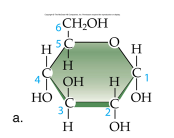Identify this molecule:

A. amino acid

B. hydrocarbon

C. carbohydrate

D. alcohol

E. lipid

C

2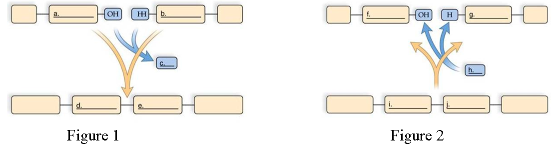Choose the Figure that depicts polymer synthesis.

Figure 1

Figure 2

Figure 1

3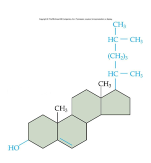Identify the following molecule:

A. amino acid

B. hydrocarbon

C. carbohydrate

D. alcohol

E. cholesterol

E

4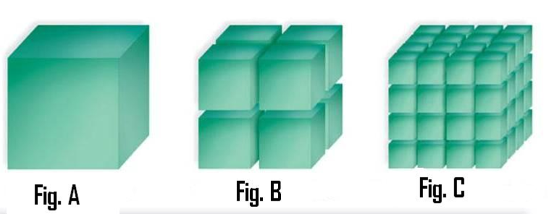Use Figures A, B and C to answer Questions 40 - 44. Figure A is an 8-cm. cube. Figure B is eight, 4-cm cubes. Figure C is sixty-four, 2-cm cubes.

What figure has the greatest surface area : volume ratio?

A. Figure A

B. Figure B

C. Figure C

D. All of these are the same.

C

5Use Figures A, B and C to answer Questions 40 - 44. Figure A is an 8-cm. cube. Figure B is eight, 4-cm cubes. Figure C is sixty-four, 2-cm cubes.

What Figure has the greatest volume (height x width x length x number of cubes)?

A. Figure A

B. Figure B

C. Figure C

D. All of these have the same volume.

D

6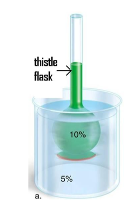A 10% glucose solution is placed in the thistle tube. The thistle tube is placed in a beaker that contains a 5% glucose solution. Where is the highest concentration of water found?

A. In the 10% solution

B. In the 5% solution

C. The concentration of water is the same in both solutions.

D. None of the above

B

7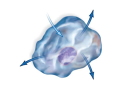In the cell pictured, there is no net movement of water. The amount leaving the cell and entering the cell is the same. In what type of environment is this cell found?

A. hypertonic

B. hypotonic

C. isotonic

D. none of the above

C

8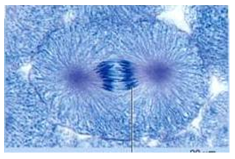What phase of mitosis is pictured?

A. prophase

B. prometaphase

C. metaphase

D. anaphase

E. telophase

D

9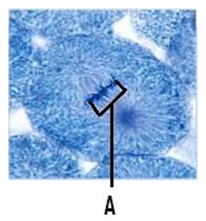What is (are) the structures designated by the letter ‘A'.

A. spindle fibers

B. centrioles

C. chromosomes

D. kinetochore microtubules

C

10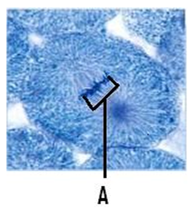What phase of mitosis is pictured?

A. prophase

B. prometaphase

C. metaphase

D. anaphase

E. telophase

C

11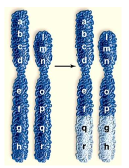The following picture depicts which of the following changes in chromosome structure.

A. deletion

B. duplication

C. translocation

D. aneuploidy

C

12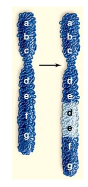The following picture depicts which of the following changes in chromosome structure.

A. deletion

B. duplication

C. translocation

D. aneuploidy

B

13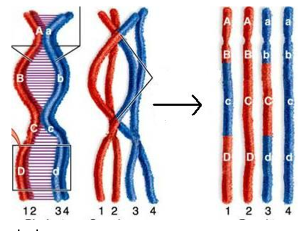Study the diagram to answer the following questions.

What process is occurring in this diagram?

A. fertilization

B. mitosis

C. crossing under

D. crossing over

D